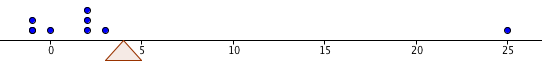﻿ Mean, Median, and Mode

#Mean, Median and Mode

You may want to review:   Summation Notation

Statistics is the discipline devoted to organizing, summarizing, and drawing conclusions from data.

Given a collection of data, it is often convenient to come up with a single number that somehow describes its center; a number that in some way is representative of the entire collection. Such a number is called a measure of central tendency.

The two most popular measures of central tendency are the mean and the median. Another measure sometimes used to describe a ‘typical’ data value is the mode.

## The Mean of a Data Set

The mean (or average) is already familiar to you: add up the numbers, and divide by how many there are:

DEFINITION mean, average
The mean (or average) of the $\,n\,$ data values $$\,\cssId{s14}{x_1,\ x_2,\ x_3,\ \ldots,\ x_n}\,$$ is denoted by $\,\bar{x}\,$ (read as ‘$\,x\,$ bar’) and is given by the formula: $$\cssId{s19}{\bar{x}}\ \cssId{s20}{= \frac{x_1 + x_2 + x_3 + \cdots + x_n}{n}}$$

or

$$\begin{gather} \bar{x} \cssId{s21}{= \frac{\sum_{i=1}^n\ x_i}{n}}\cr \cssId{s22}{\text{(using summation notation)}} \end{gather}$$

or

$$\bar{x} \cssId{s23}{= \frac1n\sum_{i=1}^n\ x_i}$$

(an alternative version of summation notation)

Thus, to find the mean of $\,n\,$ data values, you add them up and then divide by $\,n\,.$

Similarly, the mean of the $\,n\,$ data values $$\cssId{s27}{y_1,\ y_2,\ y_3,\ \ldots,\ y_n}$$ would be denoted by $\,\bar{y}\,$ and read as ‘$\,y\,$ bar’.

Since dividing by $\,n\,$ is the same as multiplying by $\,\frac{1}{n}\,,$ the notation $$\cssId{s33}{\frac{\sum_{i=1}^n\ x_i}{n}}$$ is more commonly written as: $$\cssId{s35}{\textstyle\frac 1n\sum_{i=1}^n\ x_i}$$ or $$\cssId{s36}{\frac 1n\sum_{i=1}^n\ x_i}$$

## Example: Mean

Find the mean of these data values:

$$\cssId{s39}{2,\ -1,\ 2,\ 3,\ 0,\ 25,\ -1,\ 2}$$

There are $\,8\,$ data values. The mean is found by adding them up and then dividing by $\,8\,$:

\begin{align} &\cssId{s42}{\frac{2+(-1)+2+3+0+25+(-1)+2}{8}}\cr &\qquad \cssId{s43}{= \frac{32}{8}} \cssId{s44}{= 4} \end{align} As discussed in Average of Three Signed Numbers, the mean gives the balancing point for the distribution, in the following sense: if eight pebbles of equal weight are placed on a ‘number line see-saw’ with
• two pebbles at $\,-1$
• one pebble at $\,0$
• three pebbles at $\,2$
• one pebble at $\,3$
• and one pebble at $\,25$

then the support would have to be placed at $\,4\,$ for the see-saw to balance perfectly!Notice in the previous example that the number $\,25\,$ seems to be unusually large, compared to the other numbers. An outlier is an unusually large or small observation in a data set.

A drawback of the mean is that its value can be greatly affected by the presence of even a single outlier. If the outlier $\,25\,$ is changed to $\,250\,,$ then the new mean would be $\,32.125\,,$ which does not seem at all representative of a ‘typical’ number in this data set!

## The Median of a Data Set

The median, on the other hand, is quite insensitive to outliers.

Just as the median strip of a highway goes right down the middle, the median of a set of numbers goes right through the middle of the ordered list.

Of course, only lists with an odd number of values have a true middle: the middle number in the ordered list ‘$\,5,\ 7,\ 20\,$’ is $\,7\,.$ See how the definition below solves the problem when there are an even number of data values:

DEFINITION median

To find the median of a set of $\,n\,$ data values, first order the observations from least to greatest (or greatest to least).

If $\,n\,$ is odd, then the median is the number in the exact middle of the ordered list. That is, the median is the data value in position $\,\frac{n+1}{2}\,$ of the ordered list.

If $\,n\,$ is even, then the median is the average of the two middle members of the ordered list. That is, the median is the average of the data values in positions $\,\frac{n}{2}\,$ and $\,\frac{n}{2}+1\,$ of the ordered list.

## Example: Median

Question:  Find the median of these data values: $$\cssId{s80}{2,\ -1,\ 2,\ 3,\ 0,\ 25,\ -1,\ 2}$$ (This is the same data set as in the previous example.)
Solution:   Begin by ordering the eight data values from least to greatest: $$\begin{gather} \cssId{s84}{\underset{\text{position 1}}{\underset{\uparrow}{-1,\strut}}}\ \ \ \ \cssId{s85}{\underset{\text{position 2}}{\underset{\uparrow}{-1,\strut}}}\ \ \ \ \cssId{s86}{\underset{\text{position 3}}{\underset{\uparrow}{0,\strut}}}\cr\cr \cssId{s87}{\overset{\text{the two ‘middle’ members}} {\ \ \overbrace{ \underset{\text{position 4}}{\underset{\uparrow}{2,\strut}}\ \ \ \ \underset{\text{position 5}}{\underset{\uparrow}{2,\strut}} }}}\cr\cr \cssId{s88}{\underset{\text{position 6}}{\underset{\uparrow}{2,\strut}}}\ \ \ \ \cssId{s89}{\underset{\text{position 7}}{\underset{\uparrow}{3,\strut}}}\ \ \ \ \cssId{s90}{\underset{\text{position 8}}{\underset{\uparrow}{25\strut}}} \end{gather}$$

There are an even number of values, so we average the values in positions four and five: the median is $\,\frac{2+2}{2} = 2\,.$

Note that, for this data set, the median seems to do a better job than the mean in representing a ‘typical’ member. Note also that if the outlier $\,25\,$ is changed to $\,250\,,$ it doesn't affect the median at all!

## The Mode of a Data Set

Finally, a mode is a value that occurs ‘most often’ in a data set. Whereas a data set has exactly one mean and median, it can have one or more modes.

## Examples: Mode

For example, consider these data values:

$$\cssId{s100}{2,\ -1,\ 2,\ 3,\ 0,\ 25,\ -1,\ 2}$$

Re-group them occurring to their frequency:

\begin{align} \cssId{s102}{2,\ \ 2,\ \ 2}\quad &\cssId{s103}{\text{(three occurrences of the number 2)}}\\ \cssId{s104}{-1,\ \ -1}\quad &\cssId{s105}{\text{(two occurrences of the number -1)}}\\ \cssId{s106}{0}\quad &\cssId{s107}{\text{(one occurrence of the number 0)}}\\ \cssId{s108}{3}\quad &\cssId{s109}{\text{(one occurrence of the number 3)}}\\ \cssId{s110}{25}\quad &\cssId{s111}{\text{(one occurrence of the number 25)}} \end{align}

The mode of this data set is $\,2\,,$ since this data value occurs three times, and this is the most occurrences of any data value.

Every member of the data set ‘$\,3,\ 7,\ 9\,$’ is a mode, since each value occurs only once.

The data set ‘$\,3,\ 3,\ 7,\ 7,\ 9\,$’ has two modes: $\,3\,$ and $\,7\,.$ Each of these numbers occurs twice, and no number occurs more than two times.